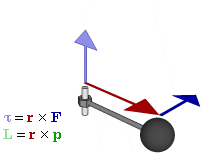# 10.16: Torque and Angular Acceleration

$$\newcommand{\vecs}{\overset { \rightharpoonup} {\mathbf{#1}} }$$ $$\newcommand{\vecd}{\overset{-\!-\!\rightharpoonup}{\vphantom{a}\smash {#1}}}$$$$\newcommand{\id}{\mathrm{id}}$$ $$\newcommand{\Span}{\mathrm{span}}$$ $$\newcommand{\kernel}{\mathrm{null}\,}$$ $$\newcommand{\range}{\mathrm{range}\,}$$ $$\newcommand{\RealPart}{\mathrm{Re}}$$ $$\newcommand{\ImaginaryPart}{\mathrm{Im}}$$ $$\newcommand{\Argument}{\mathrm{Arg}}$$ $$\newcommand{\norm}{\| #1 \|}$$ $$\newcommand{\inner}{\langle #1, #2 \rangle}$$ $$\newcommand{\Span}{\mathrm{span}}$$ $$\newcommand{\id}{\mathrm{id}}$$ $$\newcommand{\Span}{\mathrm{span}}$$ $$\newcommand{\kernel}{\mathrm{null}\,}$$ $$\newcommand{\range}{\mathrm{range}\,}$$ $$\newcommand{\RealPart}{\mathrm{Re}}$$ $$\newcommand{\ImaginaryPart}{\mathrm{Im}}$$ $$\newcommand{\Argument}{\mathrm{Arg}}$$ $$\newcommand{\norm}{\| #1 \|}$$ $$\newcommand{\inner}{\langle #1, #2 \rangle}$$ $$\newcommand{\Span}{\mathrm{span}}$$$$\newcommand{\AA}{\unicode[.8,0]{x212B}}$$

learning objectives

• Express the relationship between the torque and the angular acceleration in a form of equation

Torque and angular acceleration are related by the following formula where is the objects moment of inertia and αα is the angular acceleration.

Torque, Angular Acceleration, and the Role of the Church in the French Revolution: Why do things change their angular velocity? Soon, you’ll know.

Just like Newton’s Second Law, which is force is equal to the mass times the acceleration, torque obeys a similar law. If you replace torque with force and rotational inertia with mass and angular acceleration with linear acceleration, you get Newton’s Second Law back out. In fact, this equation is Newton’s second law applied to a system of particles in rotation about a given axis. It makes no assumptions about constant rotational velocity.

The net torque about an axis of rotation is equal to the product of the rotational inertia about that axis and the angular acceleration, as shown in Figure 1.Figure 1: Relationship between force (F), torque (τ), momentum (p), and angular momentum (L) vectors in a rotating system

Similar to Newton’s Second Law, angular motion also obeys Newton’s First Law. If no outside forces act on an object, an object in motion remains in motion and an object at rest remains at rest. With rotating objects, we can say that unless an outside torque is applied, a rotating object will stay rotating and an object at rest will not begin rotating.

If a turntable were spinning counter clockwise (when viewed from the top), and you applied your fingers to opposite sides the turntable would begin to slow its spinning. From a translational viewpoint, at least, there would be no net force applied to the turntable. The force that points to one side would be cancelled by the force that points to the other. The forces of the two fingers would cancel. Therefore, the turntable would be in translational equilibrium. Despite that, the rotational velocity would be decreased meaning that the acceleration would no longer be zero. From this we might conclude that just because a rotating object is in translational equilibrium, it is not necessarily in rotational equilibrium.

## Key Points

• When a torque is applied to an object it begins to rotate with an acceleration inversely proportional to its moment of inertia.
• This relation can be thought of as Newton’s Second Law for rotation. The moment of inertia is the rotational mass and the torque is rotational force.
• Angular motion obeys Newton’s First Law. If no outside forces act on an object, an object in motion remains in motion and an object at rest remains at rest.

## Key Terms

• angular acceleration: The rate of change of angular velocity, often represented by α.
• torque: A rotational or twisting effect of a force; (SI unit newton-meter or Nm; imperial unit foot-pound or ft-lb)
• rotational inertia: The tendency of a rotating object to remain rotating unless a torque is applied to it.